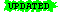CSE 111, Fall 2004

Turing Machine Program for Conjunction

 Last Update: 3 October 2004 Note:ormaterial is highlighted

I/O behavior:

Input: The truth values of two propositions, xy.
Output: The truth value of their conjunction, x and y

Input representation:

A 2-square tape, where s1 has "0" or "1" representing the truth value of x, and where s2 has "0" or "1" representing the truth value of y.

Output representation:

A 3-square tape, with s1, s2 as above, and where s3 has "0" or "1" representing the truth value of x and y

1. START; {go to state M0}

2. Keep doing whichever of the following you can:

1. if state = M0 and scanning 0 then
begin
RIGHT;
go to state M1
end;

2. if state = M0 and scanning 1 then
begin
RIGHT;
go to state M2
end;

3. if state = M1 and scanning 0 then{the actual symbol scanned is irrelevant! Why?}
begin
RIGHT;
go to state M4
end;

4. if state = M1 and scanning 1 then{the actual symbol scanned is irrelevant! Why?}
begin
RIGHT;
go to state M4
end;

5. if state = M2 and scanning 0 then
begin
RIGHT;go to state M4{not "M3", as mistakenly shown earlier!}
end;

6. if state = M2 and scanning 1 then
begin
RIGHT;
go to state M3
end;

7. if state = M3 and scanning 0 then{the TM must be scanning 0! Why?}
begin
PRINT-1;
go to state M4
end;{It is not possible to be in state M3 and scanning 1! Why?}
{However, we could have an instruction for that case, perhaps like this:}

{g'. if state = M3 and scanning 1 then ERROR STOP;}

3.STOP.